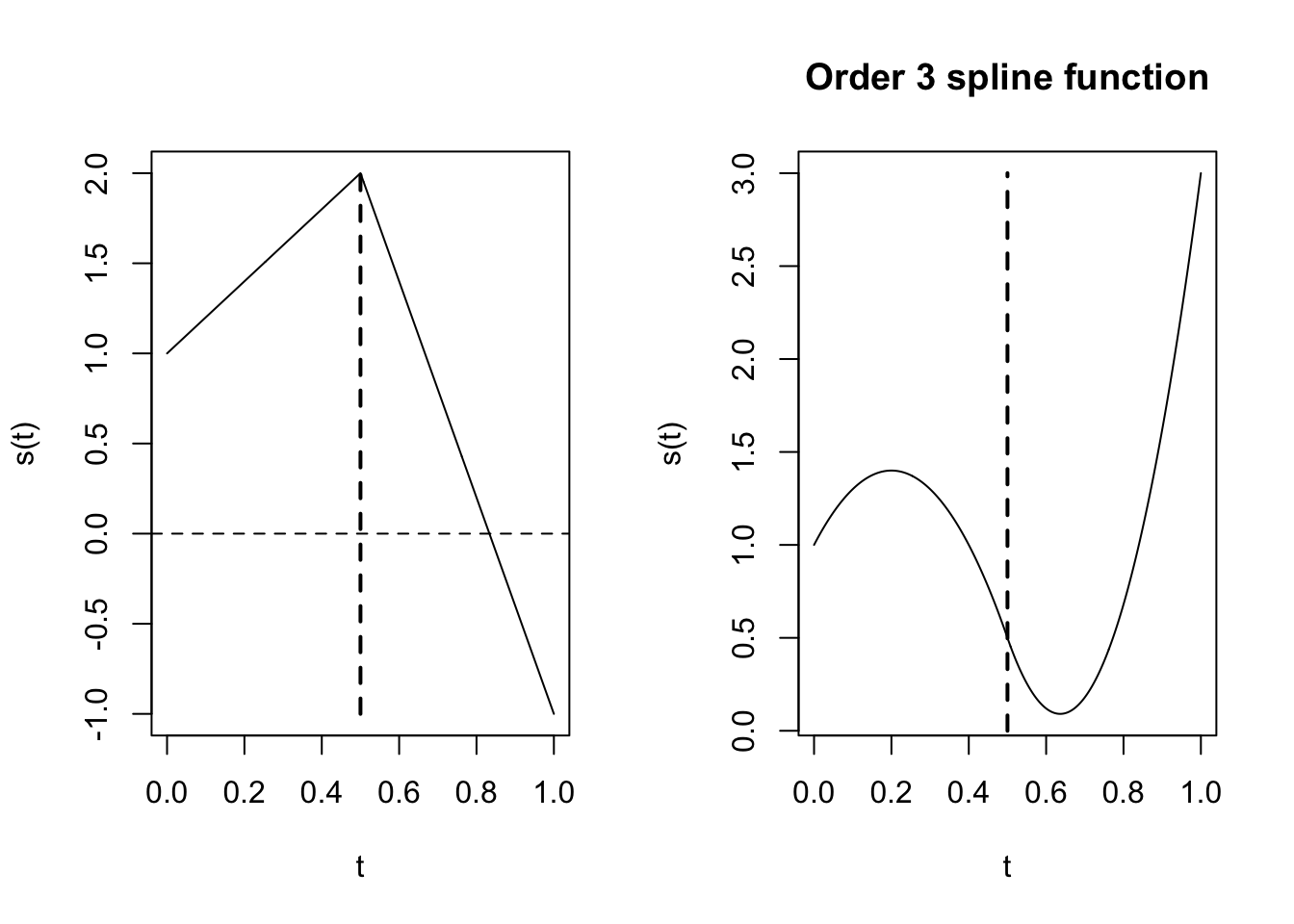library(fda)

# What is a spline function?

Expertise: Intermediate (First calculus course)

We need to know what the essential characteristics of splines are before we consider how to construct a basis system for them.

Spline functions are formed by joining polynomials together at fixed points called knots, or sometimes breakpoints. (There is a distinction between these two terms, but we will come to this later.) That is, we divide the interval extending from lower limit $$t_L$$ to upper limit $$t_U$$ over which we wish to approximate a curve into $$L+1$$ sub-intervals separated by $$L$$ interior boundaries $$\xi_\ell$$.

Consider the simplest case in which a single breakpoint divides interval [$$t_L$$; $$t_U$$] into two subintervals, as shown in the figure below The spline function is, within each interval, a polynomial of specified degree (the highest power defining the polynomial) or order (the number of coefficients defining the polynomial, which is one more than its degree). Let’s use $$m$$ to designate the order of the polynomial, so that the degree is $$m - 1$$:

At the interior breakpoint $$\xi_1$$, the two polynomials are required to join smoothly. In the most common case, this means that the derivative values on either side of the knot are equal up to the order one less than the degree. In fact, if they matched up to the derivative whose order equaled the degree, they would be the same polynomial. Consequently, a spline function defined in this way has one extra degree of freedom than a polynomial extending over the entire interval.

For example, let each polynomial be a straight line segment, and therefore of degree one. In this case, they join at the breakpoint with matching derivatives up to degree 0; in short, they simply join and have identical values at the break point. Since the first polynomial has two degrees of freedom (slope and intercept), and the second, having its value already defined at the break point, is left with only one degree of freedom (slope), the total polygonal line has three degrees of freedom.

Correspondingly, if both polynomials are quadratics, then they match both in terms of values and in terms of slope of first derivative at $$\xi_1$$. The first polynomial has three degrees of freedom, but the second loses two because if the constraints on its value and slope at $$\xi_1$$, and thus retains only one. This leaves a total of four degrees of freedom for the spline function formed in this way, as opposed to three for a quadratic polynomial over the entire interval [$$t_L$$; $$t_U$$]. Figure 1 shows these linear and quadratic cases with a single breakpoint.

par(mfrow=c(1,2))
# first graph: order 2
# set range, number of basis functions and order
rng    <- c(0,1)
nbasis <- 3
norder <- 2
#  make the spline basis object
basis2 <- create.bspline.basis(rng, nbasis, norder)
#  define three coefficients
coefs2 <- matrix(c(1,2,-1), nbasis,1)
#  make the spline function object
splfd2 <- fd(coefs2, basis2)
#  plot the spline function with a vertical dashed
#  line at the interior knot location
plot(splfd2, xlab="t", ylab="s(t)")
#>  "done"
lines(c(0.5,0.5), c(-1,2), lwd=2, lty=2)
# second graph: order 3
# set range, number of basis functions and order
rng    <- c(0,1)
nbasis <- 4
norder <- 3
#  make the spline basis object
basis3 <- create.bspline.basis(rng, nbasis, norder)
#  define four coefficients
coefs3 <- matrix(c(1,2,-1,3), nbasis,1)
#  make the spline function object
splfd3 <- fd(coefs3, basis3)
#  plot the spline function with a vertical dashed
#  line at the interior knot location
plot(splfd3, xlab="t", ylab="s(t)", main="Order 3 spline function")
#>  "done"
lines(c(0.5,0.5), c(0,3), lwd=2, lty=2)Notice that the curve segments have equal values at the knot for both plots, but that the order three (quadratic) segments also have equal slopes. Hence the higher the order, the smoother curve.

What are some examples of commonly used basis functions? We can now generalize the situation to L interior breakpoints, and a spline function being of order m or degree $$m - 1$$ over each sub-interval. The first polynomial segment has a full complement of $$m$$ degrees of freedom, but each subsequent segment has only one degree of freedom because of the $$m - 1$$ constraints on its behavior. This gives a total of $$L + m$$ degrees of freedom, or number of interior breakpoints plus the order of the polynomial segments.

Thus, spline functions are essentially generalizations of the notion of polygonal lines. They gain their flexibility in two ways: First, by the order of the polynomials from which they are built, and second, by the number of knots or breakpoints used. We usually elect to keep the order fixed, and add breakpoints as needed to get the required flexibility.

The most common order used in practice is four, and thus values of the second derivatives match at knots. If you wanted a nice smooth second derivative, you would have to go to order 5 or higher.